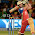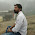# C++ Program For Infix to Postfix Expression Converter

Problem:- An infix expression is the usual way we write expressions. In infix expression, operators are written in-between their operands. For example, A * ( B + C ) / D is an infix expression. In postfix expression, operators are written after their operands. The infix expression given above is equivalent to A B C + * D /. Good Reference Article: C++ Even or Odd Program

Logic:- This program makes use of the stack. A stack is used to store elements in LIFO order. The element which comes at the end is deleted first. You can imagine that MS Word uses a stack to store operation in a specific order. When we perform ‘undo’ then it deletes the last operation performed.

This program takes a string of an infix expression and gives a string of postfix operation. It simply considers the every character of the infix string and if the character being considered is a number then it is appended to the postfix string. If it is a symbol then it is pushed onto the stack. Rest of the process is quite simple. The code is also self-explanatory.

Solution:-

#include<iostream>
#include<cstring>
using namespace std;

class stack
{
private:

int arr;
int top;
int size;
public:

stack()
{
top=-1;
size=5;
}
bool IsEmpty()
{
if(top==-1)
{
return true;
}
else
{
return false;
}
}

bool IsFull()
{
if(top==size-1)
{
return true;
}
else
{
return false;
}
}

void push(int data)
{
if(IsFull())
{
cout<<"STACK IS FULL..."<<endl;
}
else
{
top++;
arr[top]=data;
}
}

int pop()
{
if(IsEmpty())
{
cout<<"STACK IS EMPTY..."<<endl;
}
else
{
int c;
c=arr[top];
top--;
return c;
}
}

void show()
{
if(IsEmpty())
{
cout<<"STACK IS EMPTY..."<<endl;
}
else
{
for (int i=0;i<=top;i++)
{
cout<<"DATA ---- "<<arr[i]<<endl;
}
}
}

int getweight(char ch)
{
switch(ch)
{
case '/':
case '*': return 2;
case '+':
case '-': return 1;
default: return 0;
}
}

void in2p(char in[],char po[],int size)
{
int i=0,k=0;
int w;
char ch;

while(i<size)
{
ch=in[i];
w=getweight(ch);

if(w==0)
{
po[k++]=ch;
}
else
{
if(IsEmpty())
{
push(ch);
}

else
{
while(!IsEmpty() && w<=getweight(arr[top]))
{
po[k++]=arr[top];
pop();
}
push(ch);
}
}
i++;
}
while(!IsEmpty())
{
po[k]=arr[top];
k++;
pop();
}
po[k]=0;
}
};

int main()
{
char given[]="1+2*3";
int s=strlen(given);
char desired[s];
stack obj;
obj.in2p(given,desired,s);
cout<<"\n\n\n\t\t----------------------------------------------";
cout<<"\n\t\t|                                            |";
cout<<"\n\t\t|   INFIX TO POSTFIX EXPRESSION CONVERTER    |";
cout<<"\n\t\t|                                            |";
cout<<"\n\t\t----------------------------------------------\n";
cout<<"\t\tINFIX EXPRESSION = "<<given;
cout<<"\n\n\t\tPOSTFIX EXPRESSION = "<<desired<<endl<<endl;
system("pause");
};

1.how to make my programming very perfect

1.if you want to perfect in programming then you have to choose any online editor like geeksforgeeks and hacker rank, and code on it.
Make sure you start with ground (Zero) level and daily practice. for example today if you choose array after choosing today topics practice all problem related to your topic and next day choose another and again repeat same process. Or you can direct contact me i will explain daily plan for you day by day, and also monitor your progress this will help to improve your coding skill instantly.

Thanks for visit keep sharing. Happy coding

2.It is very interesting but additional I want that the code accept any mathematical functions such as log(), abs(), sin(), cos(), and sqrt() and calculate the result Ex. (5+4)*(log(2)-77.9) ) = -698.3

Could possible to help me with that?

1.Hi, You can easily calculate these mathematical functions cause these all are already implements in C++, this is a simple example of "Infix to Postfix Expression Converter" and these conversion simply follow BODMAS rules or simply you can say if an expression contains brackets ((), {}, []) we have to first solve or simplify the bracket followed by of (powers and roots etc.), then division, multiplication, addition and subtraction from left to right.

Thanks